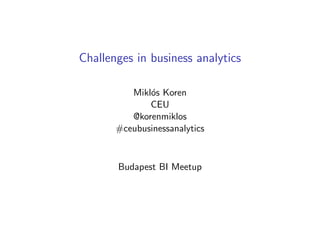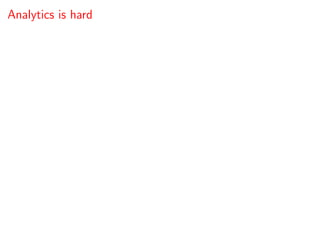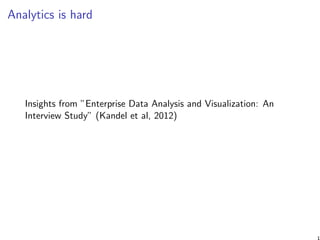Successfully reported this slideshow.

# Challenges in business analytics×

1 of 16 Ad
1 of 16 Ad

# Challenges in business analytics

I present the results of an interview study on what type of problems real-life business analysts face and show how CEU's new MSc program helps overcome these problems.

I present the results of an interview study on what type of problems real-life business analysts face and show how CEU's new MSc program helps overcome these problems.

### Challenges in business analytics

1. 1. Challenges in business analytics Mikl´os Koren CEU @korenmiklos #ceubusinessanalytics Budapest BI Meetup
2. 2. Analytics is hard
3. 3. Analytics is hard Insights from ”Enterprise Data Analysis and Visualization: An Interview Study” (Kandel et al, 2012) 1
4. 4. ”I spend more than half of my time integrating, cleansing and transforming data without doing any actual analysis.” 2
5. 5. ”In practice it tends not to be just data prep, you are learning about the data at the same time, you are learning about what as- sumptions you can make.” 3
6. 6. ”We meet every week, we ﬁnd some interesting insight, and we say that’s great. There’s no repeatable knowledge, so we end up repeating the process 1 year later.” 4
7. 7. ”It is really hard to know where the data is. We have all the data, but there is no huge schema where we can say this data is here and this variable is there. ... It’s more like folklore. Knowledge is transmitted as you join.”
8. 8. ”Diversity is pretty important. A generalist is more valuable than a specialist. A specialist isn’t ﬂuid enough. We look for pretty broad skills and data passion.” 6
9. 9. ”Run a Hadoop job, then run a Hadoop job on results, then awk it... Hadoop job chained to Hadoop job chained to a Python script to actually process data.” 7
10. 10. Why is analytics hard? Statistics is hard. People with diﬀerent skills interact. Multiplicity of tools. (Natural for a fast evolving technology.) Implementing a business analytics project is subject to the economics of technology adoption. 8
11. 11. Why is analytics hard? Statistics is hard. People with diﬀerent skills interact. Multiplicity of tools. (Natural for a fast evolving technology.) Implementing a business analytics project is subject to the economics of technology adoption. ”Hard” pays oﬀ (Strata survey 2013).
12. 12. Hackers, scripters and users Application UserScripterHacker Analytics BiologyDatam art FinanceFinanceHealthcare Healthcare Healthcare Healthcare Insurance M arketing M arketing NewsAggregator RetailRetailSocialNetworking SocialNetworking SocialNetworking Visualization Visualization W ebW eb Analytics Analytics Analytics FinanceHealthcare M ediaRetail Finance Insurance RetailRetailSportsW eb Security DiscoveryLocating Data x x x x x x x x x x x x x x x x x Field Definitions x x x x x x x x x x x x x x x x Wrangle Data Integration x x x x x x x x x x x x x x x x x x x x x x x Parsing Semi-Structued x x x x x x x x x x x x x x x x x x x x x x x Advanced Aggregation and Filtering x x x x x x x x x x x x x x x x Profile Data Quality x x x x x x x x x x x x x x x x x x x x x x x x x x x x x x Verifying Assumptions x x x x x x x x x x x x x x x x x x x x x x x x Model Feature Selection x x x x x x x x x x x x x x x x x x x x Scale x x x x x x x x x x x x x x x x x x x x x x x x x x x x x x x Advanced Analytics x x x x x x x x x x x x x x Report Communicating Assumptions x x x x x x x x x x x x x x x x x Static Reports x x x x x x x x x x x x x x x x x Workflow Data Migration x x x x x x x x x x x x x x x x Operationalizing Workflows x x x x x x x x x x x x x x x x Database SQL x x x x x x x x x x x x x x x x x x x x x x x x x x x Hadoop/Hive/Pig x x x x x x x MongoDB x x CustomDB x x x x x x x Scripting Java x x x x x x x x x x x x x Perl x x Python x x x x x x x x x x x x x x x Clojure x Visual Basic x x x Modeling R x x x x x x x x x x x x x x x x Matlab x x x x x x x SAS x x x x x Excel x x x x x x x x x x x x x x x x x x x x x ProcessTools Fig. 1. Respondents, Challenges and Tools. The matrix displays interviewees (grouped by archetype and sector) and their corresponding chal- lenges and tools. Hackers faced the most diverse set of challenges, corresponding to the diversity of their workﬂows and toolset. Application users and scripters typically relied on the IT team to perform certain tasks and therefore did not perceive them as challenges. Hackers reported using a variety of tools for visualization, includ- ing statistical packages, Tableau, Excel, PowerPoint, D3, and Raph¨ael. Six hackers viewed tools that produce interactive visualizations as re- porting tools and not exploratory analytics tools. Since they could not pivoting. I ask the IT team to pull it. Application users typically worked on smaller data sets than the other groups and generally did not export data from the spreadsheet 9
13. 13. Data scientists using more tools are paid morenumber of tools. Median base salary is constant at \$100k for those using up to 10 tools, but increases with new tools after that.9 Given the two patterns we have just examined—the relationships be‐ 10
14. 14. This calls for an interdisciplinary approach
15. 15. This calls for an interdisciplinary approach Our new program puts equal weight on 1. statistics, 2. economics, 3. management, 4. and technology. 11
16. 16. Program highlights U.S. accredited M.Sc. degree (”feln˝ottk´epz´es” in progress) 1-year program, full time or part time 10 core courses and many electives Final analytics project Industry partners: IBM, Clementine Consulting, ...